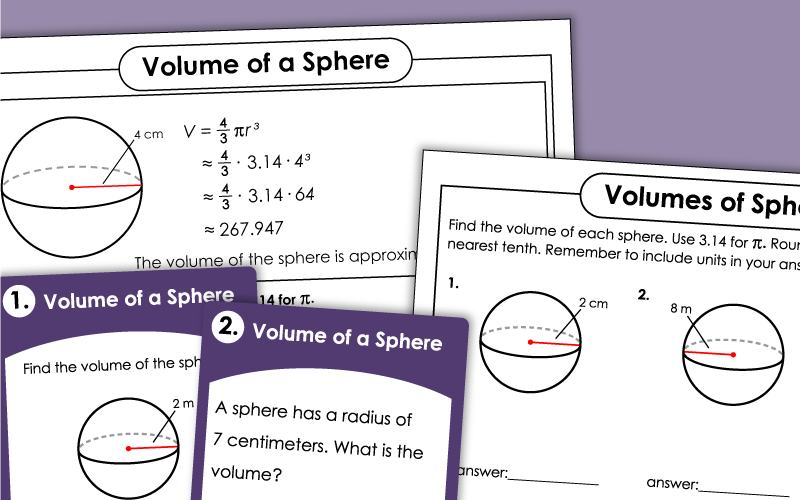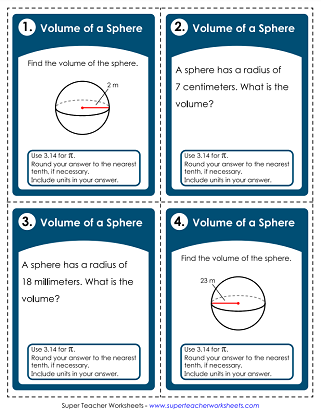# Volume of a Sphere

Practice calculating the volumes of spheres. The basic worksheets include whole number measurements for radii and require students to round to the nearest tenth. The advanced worksheets have decimal measurements for radii and require students to round to the nearest hundredth.## Level: Basic

At the top is a sample problem that shows students how to calculate the volume of a sphere. Below are six problems for students to solve.
Students are given the radius of none different spheres. They calculate the volume of each.
Print this file on card stock. Each of the 25 cards has a different problem to solve. Use for peer review sessions, on your whiteboard document camera, or classroom games.

At the top of this page is a box with a model problem, showing students how to calculate the volume of a sphere. Below are six problems for them to solve.
More Volume Worksheets

Link to other volume pages on this site. Find the volumes of cones, rectangular prisms, pyramids, cylinders, and more.

## Sample Worksheet ImagesMy Account
Site Information